# Parabolas, Rectangles, Triangles & Snakes (Part 10)

Well, this post has been a while coming (since Part 9), not because it took a lot of work. Quite the opposite, more regarding a lack of progress on this project in the last few months! Life has a way of getting in the way sometimes (by this I mean work essentially…. you know how it is… ).

A few months back I hit a bit of a roadblock, due in part to my limited knowledge of python and some annoying numerical precision issues I was having when sorting data.

I noticed some of the numerical sorting I was doing to lists were subtly changing the precision of some of the numbers. It seemed I was losing some degree of precision during certain operations which I couldn’t explain. So, the unsorted numbers could no longer be compared directly to the sorted numbers.

This was something that was most definitely required based on what I was doing (lots of sorting and loops and do this if this number from new list equals this number from old list and similar shenanigans).

But if this number doesn’t equal that number, when this number should equal that number, then shit starts going off the rails and the code starts spitting out incorrect numbers.

It first manifested itself when I was testing some stuff and I noticed the calculated centroid simply couldn’t physically be where my code was telling me it was, or random errors were occurring only when using particular combinations of inputs which triggered some numerical precision issues.

The only way the numbers can lie, is if the numbers are wrong. If the numbers are wrong, then I’ve also gone wrong by extension. Sheep 1 vs Engineer 0, dammit.

It was about this time I was all about dealing with it later as it all got too hard to track down and deal with the culprit (likely multiple culprits). The project went on the back burner for a bit longer than expected as other more pressing things like work (yawn) took over…

### More maths… yawn!

Then one day I discovered line integrals…. Like this basically…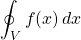I’d seen this integration squiggle + circle before during researching evaluating concrete stress blocks. But had no idea of what it meant at the time. But obviously it means the integral is a line integral.

It was one of those eureka moments where you realise all the work you’ve done to date dicing and slicing a section up into manageable chunks and finding the force and centroid of each tiny bit of the section you’re looking at could be achieved in a potentially much simpler way, and in a far more mathematically elegant way.

This leaves me wondering why I’ve never heard of them beforehand. Line integrals, where have you been all my life….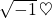maths!

I do not recall ever learning about line integrals at University. But there are lots of things I don’t remember from those years to be honest.

If you don’t use it, you tend to forget. If you get older, you forget. If you drink too much at University, you forget. You just forget, it’s life.

They say the only sure things in life are death and taxes, we’ll forgetting shit is up there to, and I’d like it officially acknowledged as the third sure thing in life. Death taxes and… er… something else?

What were we talking about again…? Yes, line integrals, what are they you might ask?

Basically if you have a line/curve which can be described via an equation (think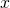&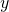coordinates) going through some vector field of which the magnitude can also be described via an equation (think the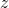coordinate), then you can do some integrating over that curve and the vector field and determine the total sum of that vector field acting on said line path.

Now to put it in context with our problem relating to compression blocks with parabolic or any other manner of stresses acting on them: –

The vector field can be equated to the stress field which varies over the depth of the compression block. We have an equation for this variation in stress if the section is orientated such that neutral axis is horizontal (parallel to the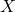axis) and the stress varies as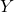varies vertically.

The line could be any line through the stress block region. For a straight line, the equation is easy to determine for example. We have the coordinates of the shape of the perimeter of the compression block so have some closed polygon coordinates travelling around the perimeter of the vector field that we are interested in.

### Green’s Thereom to the rescue!

Now at some point in history some smart chap (called George Green) produced Green’s Theorem. What Green’s Theorem basically states is if you go around the full perimeter of a closed shape in a counter clockwise direction evaluating all the piecewise line integrals over the vector field, then the sum of all these individual line integrals equates to the sum of the total vector field acting on the area/shape that’s enclosed by all the lines. Magic basically, and directly applicable to the stress block force summation we are trying to derive.

Green’s Theorem is usually stated in the following manner, where Green’s theorem transforms the double integration over an areainto a line integration along a closed curve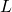that encloses the area:-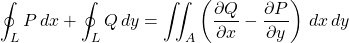Where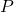and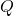are functions ofand, andis the area of interest.

Basically up to now we’ve been doing the double integral approach, though we’ve phrased it slightly differently because we wrote our integral in terms of a single variableinstead of both&.

In terms our problem of evaluating the concrete force, if our section is transformed so the neutral axis is parallel to theaxis then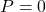. Because the vector field does not vary in thedirection, it only varies in thedirection.

If you also define the following relationship foryou can use Green’s theorem to evaluate the concrete force, and moments about both transformed axes.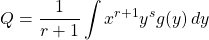Where if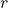andvariables are set as follows, your sum of the line integrals will evaluate either the force, or moments about either transformed axis: –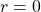,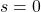evaluates the concrete force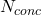.,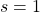evaluates the concrete moment about theaxis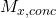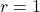,evaluates the concrete moment about theaxis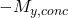, note the negative sign here.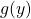is our stress function, our equation for the stress at a point. For a rectangular stress block this will equate to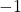, for the EC2 parabolic stress block it will be the equation out of EC2 as a ratio of maximum stress varying from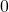to.

Of note also is that a line with constantcoordinates will result in the line integral evaluating to zero. We can make use of this fact to skip any unnecessary evaluations of our concrete stress functions (every little bit helps).

If we multiply the sum of all the individual line integrals by our ‘alpha’ factor and concrete strength from our code, then we get a direct answer for the force and moment for a given neutral axis depth. No need to evaluate centroids as its all wrapped up in the method used. This hopefully equates to a lower computational overhead and easier to read code.

Now before you think I’m some sort of genius for figuring all this out all on my own, I got some help on the process out of a pretty handy paper on the subject which explained the general processes involved and gave an example which was fairly useful for nutting it all out.

Now I will say that in my opinion there are some significant errors in the example given in the paper and some of the logic, and some parts definitely could do with a better explanation than is given. It’s like the reviewer thought all this looks pretty complicated (and over my head) so it must be right… No need to actually check it right?… Need I say more…

But not withstanding these indiscretions, the rest is good stuff once you figure it out on your own and work through the obvious errors the author made.

There’s obviously a more theoretically correct “calculus” based explanation to line integrals and Green’s theorem regarding little small infinitesimal areas and so forth and the sum of the vector field bits on the sides of these adjacent infinitesimally small areas cancelling each other out except for at the perimeter, so we sum shit around the perimeter or something and it gives you the same answer. But as I’ve said before in one of the earlier parts of this series, this isn’t an integration lesson, and my explanation clearly does not do the subject justice.

All you really need to know if you’re following along but have no idea of what’s going on, is that it’s basically a form of mathematical voodoo magic when you first stumble upon it, compared at least to the brute force method I was pursuing previously.

Understanding what the Green chap was on about proves rather useful for our problem. All those promises made by my secondary school teachers that calculus would one day become useful for something are perhaps true?

So, we have a 2D shape, being our compression block outline. We have a stress/vector field acting on that shape. Throw in a bit of numerical integration, what could possibly go wrong…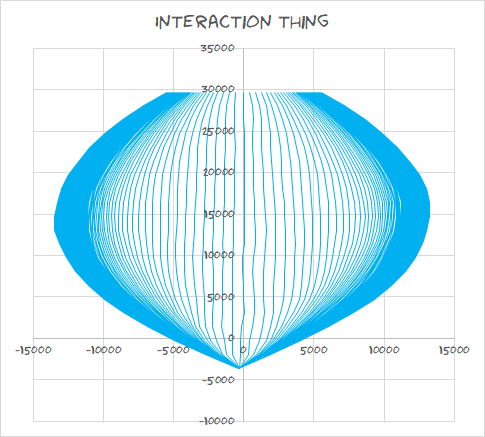mock-up/test calculations in Excel to figure out this new approach vaguely looks like it should

Now to be clear, there was nothing really wrong with the previous approach I was applying. It was just a bit messy in hindsight compared with this more elegant approach. One step forward, two steps back I guess, that’s structural engineering some most days…